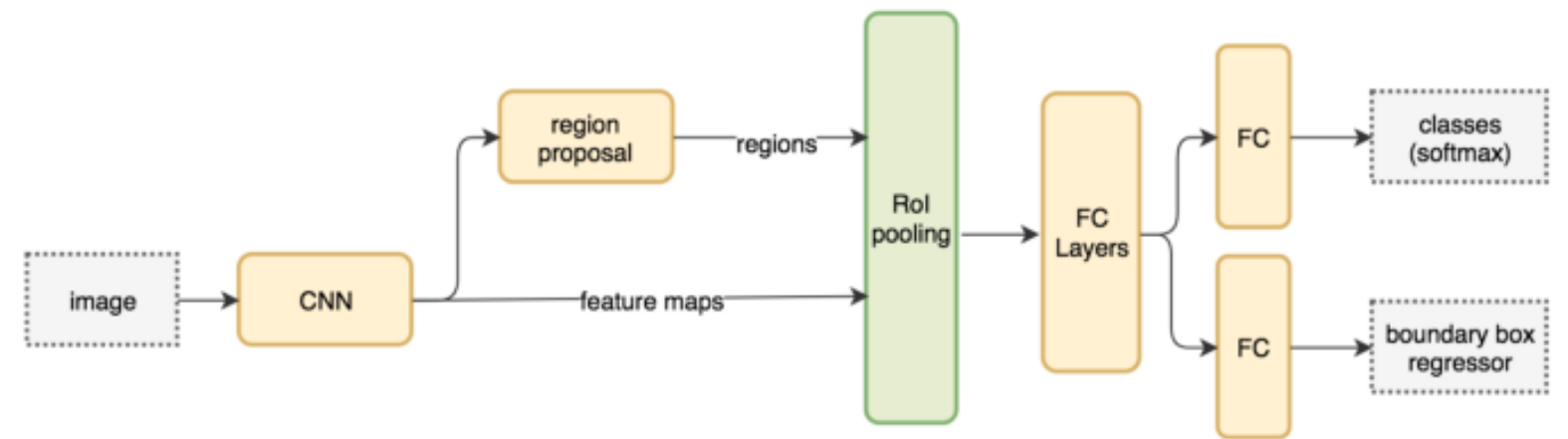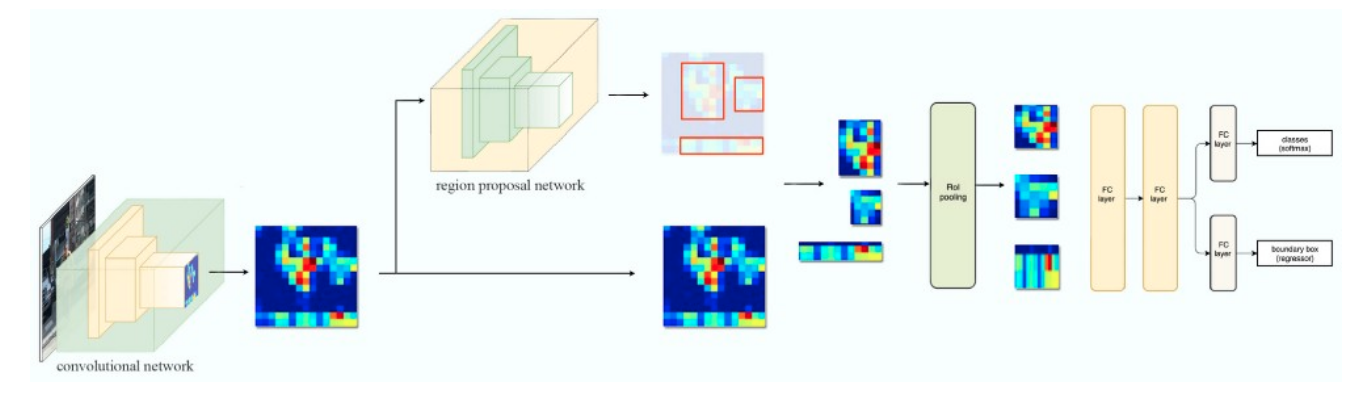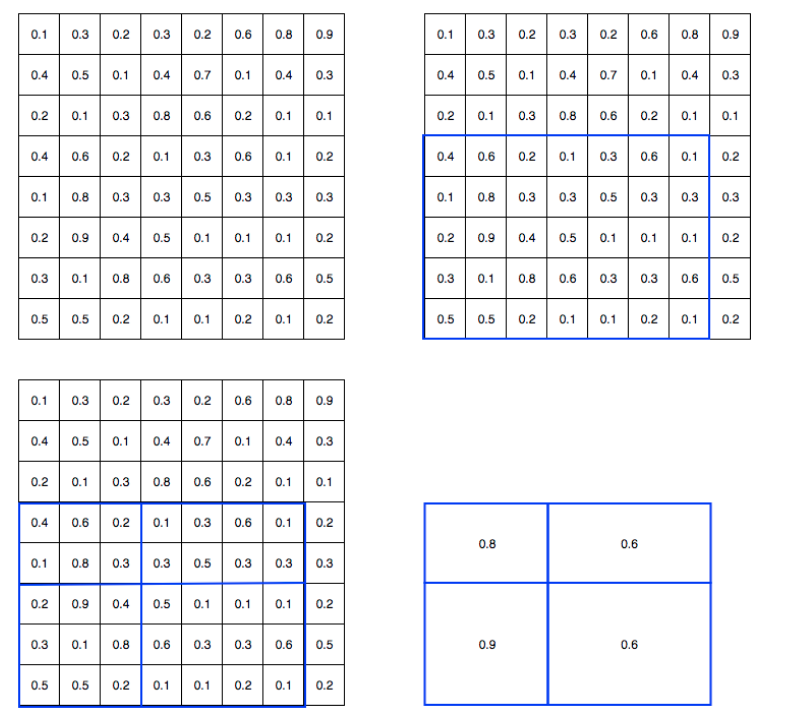@huanghaian 2020-11-18T05:54:14.000000Z 字数 4933 阅读 1605

# mmdetection：Faster RCNN深入分析

mmdetection

## 0 摘要

faster rcnn简要流程是：首先采用二分类RPN网络提取大量前后景roi特征图，然后通过roipool变成统一大小，最后将roi特征图输入到rcnn中进行roi的refine，得到优化后的bbox。

## 1 算法分析### 1.2 RPN部分

RPN层的作用是基于预设值anchor进行二分类前后景提取和bbox回归，主要目的是为rcnn层输入高质量的后续bbbox，这部分内容请看RPN算法解读部分文章。

### 1.3 RCNN

RCNN部分和RPN模块非常类似，只不过处理的问题不一样而已。整个RCNN部分的配置如下所示：

roi_head=dict(    type='StandardRoIHead',    bbox_roi_extractor=dict(        type='SingleRoIExtractor',        roi_layer=dict(type='RoIAlign', output_size=7, sampling_ratio=0),        out_channels=256,        featmap_strides=[4, 8, 16, 32]),    bbox_head=dict(        type='Shared2FCBBoxHead',        in_channels=256,        fc_out_channels=1024,        roi_feat_size=7,        num_classes=80,        bbox_coder=dict(            type='DeltaXYWHBBoxCoder',            target_means=[0., 0., 0., 0.],            target_stds=[0.1, 0.1, 0.2, 0.2]),        reg_class_agnostic=False,        loss_cls=dict(            type='CrossEntropyLoss', use_sigmoid=False, loss_weight=1.0),        loss_bbox=dict(type='L1Loss', loss_weight=1.0))))

(1) rpn和rcnn中间数据转换流程

rpn_proposal=dict(    nms_across_levels=False,    nms_pre=12000,    nms_post=2000,    max_num=2000,    nms_thr=0.7,    min_bbox_size=0),

rcnn需要的输入数据来自rpn预测roi，故在运行rcnn模块前需要对rpn输出进行处理，配置如上，这个步骤其实就等价于rpn自身的前向推理流程。具体操作是可以看RPN算法解读的前向推理部分，其大概流程是：

• 遍历每个RPN输出层(其实就是1个)，对每一层的分类分值scores进行降序排列，保留topk=nms_pre个样本，分值越大越可能是正样本
• 此时就可以收集到所有层的所有样本，先过滤掉不满足min_bbox_size预测结果，然后统一进行nms即可，最好保留最多前nms_post个检测结果

(2) roipool操作(3) RCNN网络结构

(4) 正负样本定义和采样

def forward_train(self,                  x,                  img_metas,                  proposal_list,                  gt_bboxes,                  gt_labels,                  gt_bboxes_ignore=None,                  gt_masks=None):

x是backbone输出的stride=16的特征图，img_metas是每张图片各自的属性，proposal_list就是RPN输出的roi，假设是(batch,1000,4)，后面几个都是关于gt bbox相关的数据。

RCNN算法要完成的任务是：基于RPN输出的proposal_list来回归出更好的bbox。此时可以发现RCNN算法其实和RPN思想非常类似，只不过RPN中学习目标是anchor和gt bbox的变换值，而RCNN模块可以认为proposal_list是稀疏的anchor，其学习目标是proposal_list和gt bbox的变换值。

rcnn=dict(    assigner=dict(        type='MaxIoUAssigner',        pos_iou_thr=0.5,        neg_iou_thr=0.5,        min_pos_iou=0.5,        match_low_quality=False,        ignore_iof_thr=-1),    sampler=dict(        type='RandomSampler',        num=512,        pos_fraction=0.25,        neg_pos_ub=-1,        add_gt_as_proposals=True),    pos_weight=-1,    debug=False))

• 由于rcnn在网络训练前期，rpn无法输出大量高质量样本，故为了平衡和稳定rcnn训练过程，通常会对rcnn head部分添加gt bbox作为proposal
• rcnn部分match_low_quality=False，原因是rcnn面对的正样本都是比较高质量的，没必要match_low_quality设置为True，因为其可能会导致低质量anchor匹配

(5) loss计算

### 1.4 推理流程

test_cfg = dict(    rpn=dict(        nms_across_levels=False,        nms_pre=6000,        nms_post=1000,        max_num=1000,        nms_thr=0.7,        min_bbox_size=0),    rcnn=dict(        score_thr=0.05,        nms=dict(type='nms', iou_threshold=0.5),        max_per_img=100))

(1) 对于单张图片输入到resnet中，输出1个特征图stride=16
(3) 采用rpn测试配置对预测结果进行解码，并且经过nms得到proposal_list
(4) 对每个proposal进行roipool操作，变成统一大小
(5) 组成batch输入到rcnn head进行类别分类和proposal refine回归
(6) 对预测结果再次进行nms操作得到优化后的最终bbox

## 2 总结

faster rcnn是经典的two-stage目标检测算法，其对rpn层提取的大量roi进行refine操作，可以得到精确的bbox。由于其优雅高效的实现，理解上也通俗易懂，故依然是目前的主流算法。对于初学者而言一开始接触就faster rcnn会被其复杂代码绕晕的，最好先把RPN思想彻底理解后再来看本算法，会轻松很多。当然在理解了faster rcnn后，对于后续的one-stage算法就更加容易了。• 私有
• 公开
• 删除